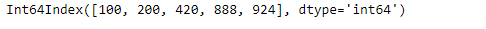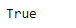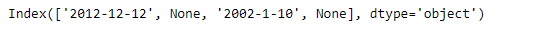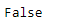# Python | Pandas Index.is_monotonic_increasing

Pandas Index is an immutable ndarray implementing an ordered, sliceable set. It is the basic object which stores the axis labels for all pandas objects.

Pandas` Index.is_monotonic_increasing` attribute return `True` if the underlying data in the given Index object is monotonically increasing else it return `False`.

Syntax: Index.is_monotonic_increasing

Parameter : None

Returns : boolean

Example #1: Use `Index.is_monotonic_increasing` attribute to find out if the underlying data in the given Index object is monotonically increasing or not.

 `# importing pandas as pd ` `import` `pandas as pd ` ` `  `# Creating the index ` `idx ``=` `pd.Index([``100``, ``200``, ``420``, ``888``, ``924``]) ` ` `  `# Print the index ` `print``(idx) `

Output :Now we will use `Index.is_monotonic_increasing` attribute to find out if the underlying data in the given Index object is monotonically increasing or not.

 `# check if the values in the Index ` `# are monotonically increasing ` `result ``=` `idx.is_monotonic_increasing ` ` `  `# Print the result ` `print``(result) `

Output :As we can see in the output, the `Index.is_monotonic_increasing` attribute has returned `True` indicating that the underlying data of the given Index object is monotonically increasing.

Example #2 : Use `Index.is_monotonic_increasing` attribute to find out if the underlying data in the given Index object is monotonically increasing or not.

 `# importing pandas as pd ` `import` `pandas as pd ` ` `  `# Creating the index ` `idx ``=` `pd.Index([``'2012-12-12'``, ``None``, ``'2002-1-10'``, ``None``]) ` ` `  `# Print the index ` `print``(idx) `

Output :Now we will use `Index.is_monotonic_increasing` attribute to find out if the underlying data in the given Index object is monotonically increasing or not.

 `# check if the values in the Index ` `# are monotonically increasing ` `result ``=` `idx.is_monotonic_increasing ` ` `  `# Print the result ` `print``(result) `

Output :As we can see in the output, the `Index.is_monotonic_increasing` attribute has returned `False` indicating that the underlying data of the given Index object is not monotonically increasing.

My Personal Notes arrow_drop_upCheck out this Author's contributed articles.

If you like GeeksforGeeks and would like to contribute, you can also write an article using contribute.geeksforgeeks.org or mail your article to contribute@geeksforgeeks.org. See your article appearing on the GeeksforGeeks main page and help other Geeks.

Please Improve this article if you find anything incorrect by clicking on the "Improve Article" button below.

Article Tags :

Be the First to upvote.

Please write to us at contribute@geeksforgeeks.org to report any issue with the above content.